# AQA A Level Chemistry复习笔记5.2.5 Finding Activation Energy

### Finding Activation Energy

#### Finding the Activation Energy

• Very often, the Arrhenius Equation is used to calculate the activation energy of a reaction
• Either a question will give sufficient information for the Arrhenius equation to be used, or a graph can be plotted and the calculation done from the plot

#### Using the equation:

• Remember, it is usually easier to use the version of the Arrhenius equation after natural logs of each side have been taken

#### Worked Example

Calculate the activation energy of a reaction which takes place at 400 K, where the rate constant of the reaction is 6.25 x 10-4 s-1.

A = 4.6 x 1013 and R = 8.31 J K-1 mol-1.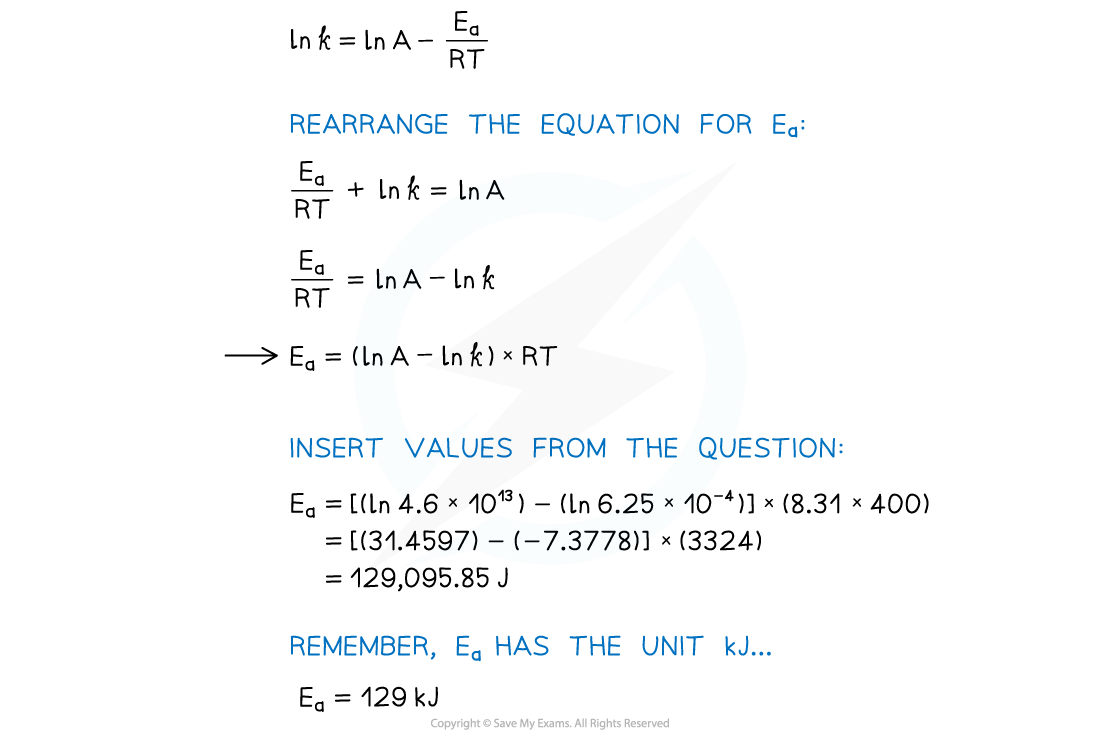#### Using an Arrhenius plot:

• A graph of ln k against 1/T can be plotted, and then used to calculate Ea
• This gives a line which follows the form y = mx + c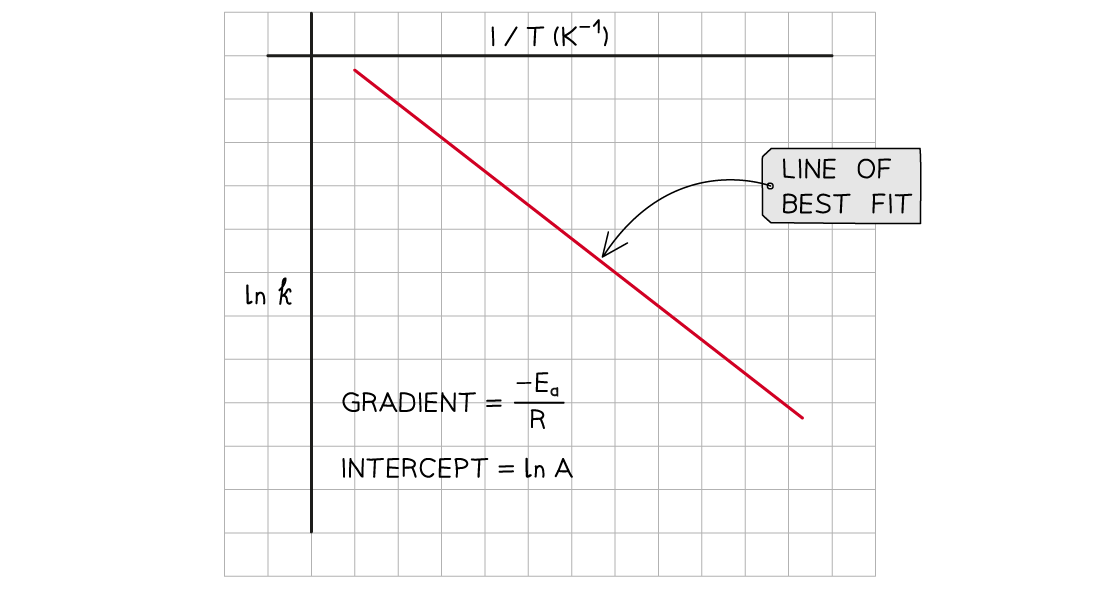The graph of ln k against 1/T is a straight line with gradient -Ea/R

• From the graph, the equation in the form of y = mx + c is as follows:#### Worked Example

Complete the following table, plot a graph of ln k against 1/T and use this to calculate the activation energy, Ea, and the Arrhenius Constant, A, of the reaction.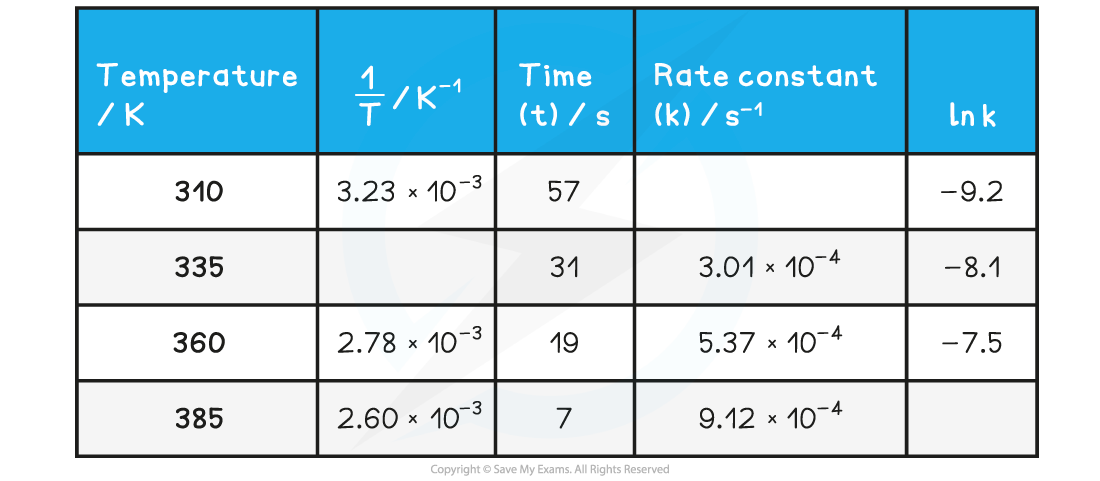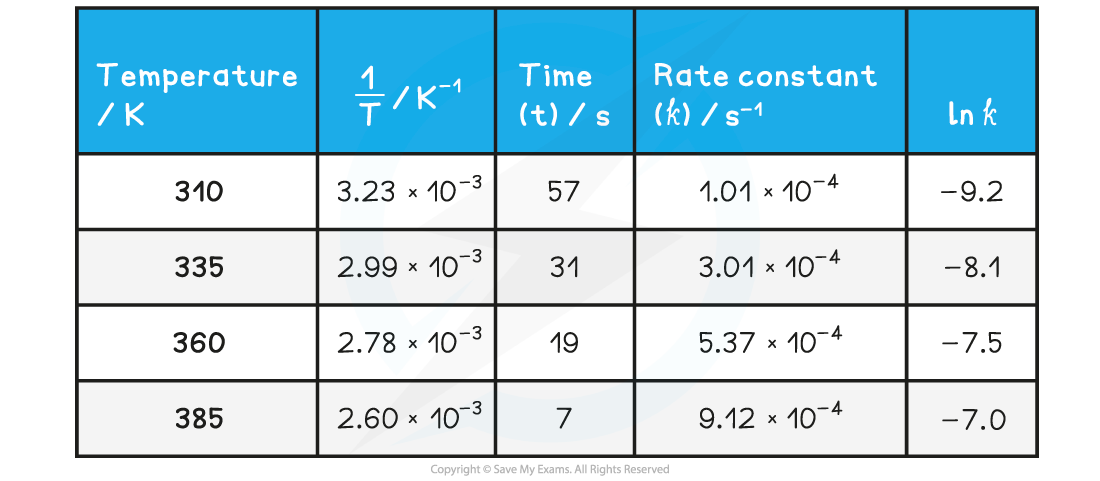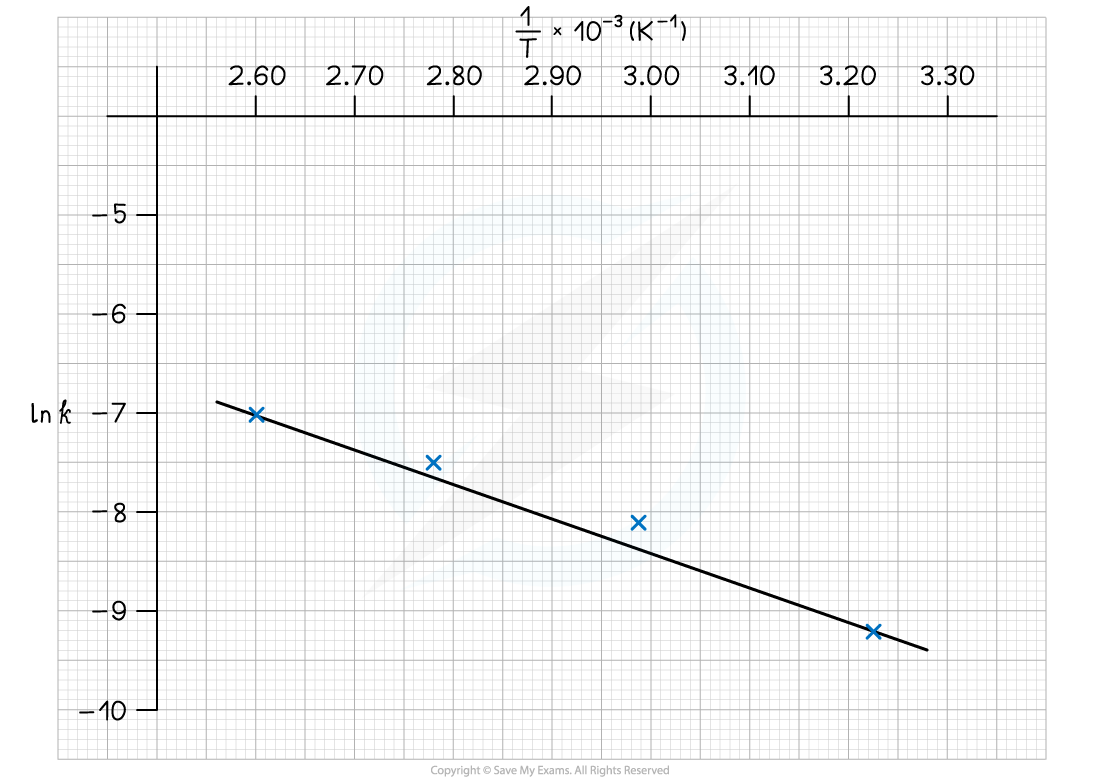Calculate the activation energy, Ea, and the Arrhenius Constant, A, of the reaction: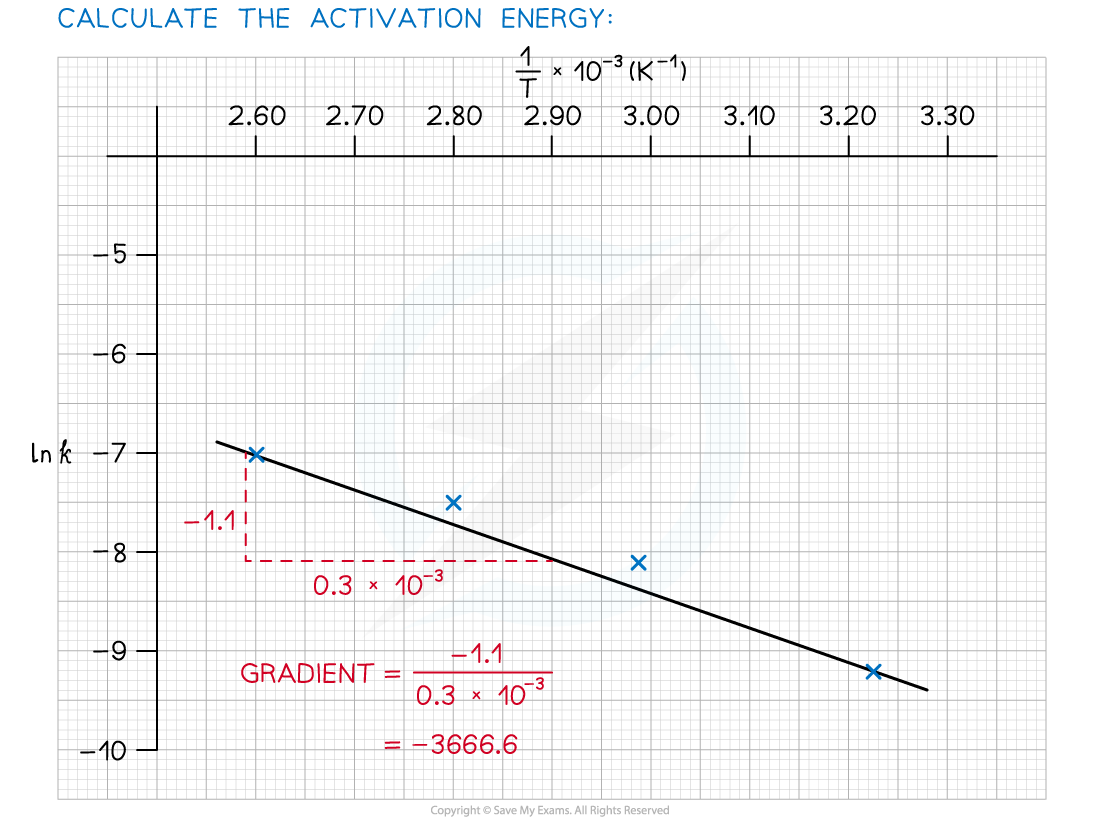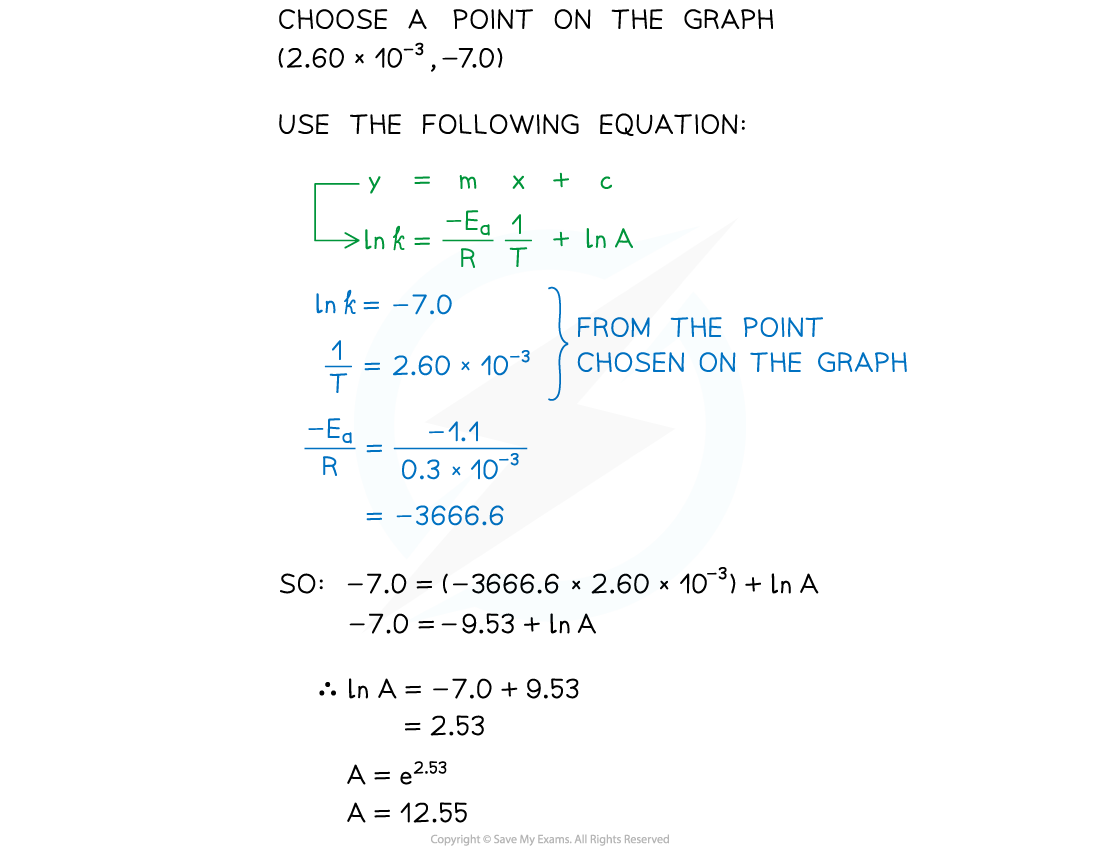#### Exam Tip

You are not required to learn these equations. However, you do need to be able to rearrange them, and knowing them is helpful in understanding the effects of temperature on the rate constant.